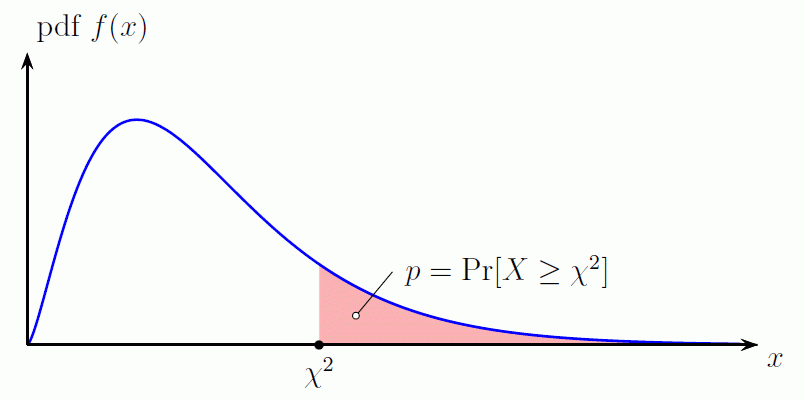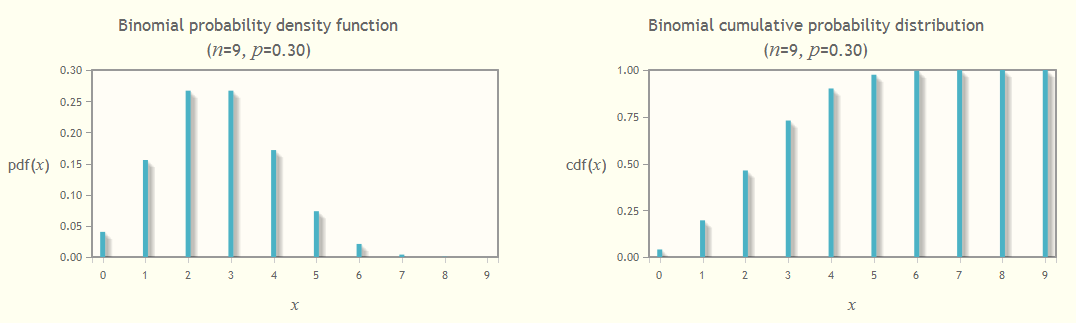DI Management Home > Mathematics > Chi-square calculator

#Chi-square calculator

To view the graph of the χ2 distribution for your calculated values, click on the show graph button after doing the calculation.

## Compute the p-value for a chi-square distribution

Affiliate disclosure: we get a small commission for purchases made through the above links

 χ2 value: (χ2 ≥ 0) degrees of freedom, ν: (ν an integer > 0) p-value = ??The p-value is the area under the chi-square probability density function (pdf) curve to the right of the specified χ2 value. In Excel: p = `CHIDIST(χ2,ν)`.

See Chi-square formulae for more details of the mathematics.

## Compute the inverse of the p-value for a chi-square distribution

 p value: (0 < p ≤ 1) degrees of freedom, ν: (ν an integer > 0) χ2 = ??

This is the value of χ2 that will give the specified p-value for the chi-square distribution. In Excel: χ2 = `CHIINV(p,ν)`.

## Table of selected percentiles

See a table of selected percentiles of the chi-square distribution computed using the Javascript calculation engine behind this page. This is the usual table we see in textbooks. You can see a comparison of our results with those given by Excel.

## Other statistical calculatorsSee our Binomial distribution calculator which calculates a table of the binomial distribution for given parameters and displays graphs of the distribution function, f(x), and cumulative distribution function, F(x).

## An aside on Post-Quantum Cryptography

Are you interested in Post-Quantum Cryptography (PQC)? Then see our pages on SPHINCS+, a stateless hash-based signature scheme chosen as one of the first quantum-resistant signature algorithms in NIST's post-quantum cryptography standardization project.

## Acknowledgements

Many thanks to Phil O'Sullivan, statistician extraordinaire, for his help and advice on this subject.